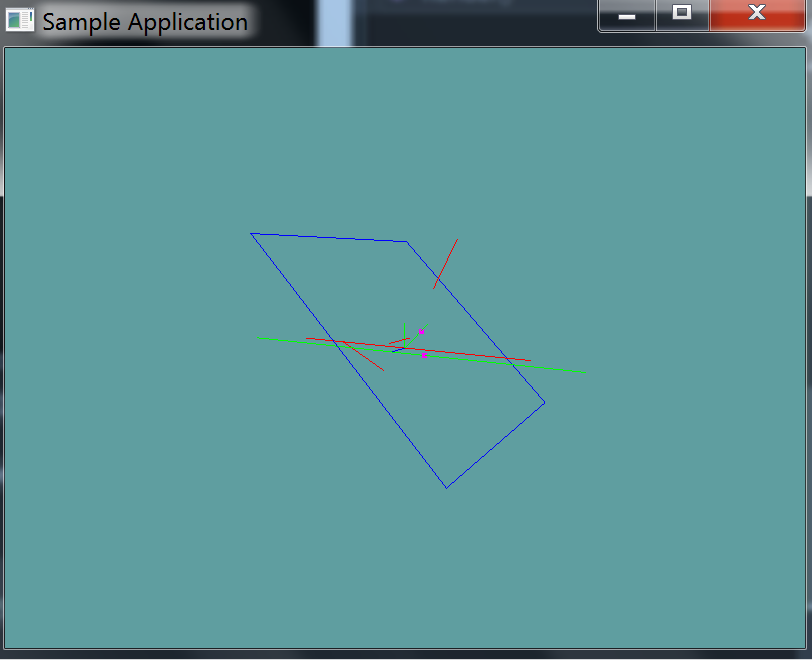# Line Against Plane

Again, this test is almost the same as the previous two. The code here should be really straight forward.

### Implementation

Provide an implementation for the following function

public static bool LineTest(Line line, Plane plane, out Point result) {


### Unit Test

You can Download the samples for this chapter to see if your result looks like the unit test.

This unit test will print out errors if any are present in your code.using OpenTK.Graphics.OpenGL;
using Math_Implementation;
using CollisionDetectionSelector.Primitives;

namespace CollisionDetectionSelector.Samples {
class LinetestPlane : Application {
public Line[] lines = new Line[] {
new Line(new Point(2.5f, 2.5f, 2.5f), new Point(4, 4, 4)), // false
new Line(new Point(1f, 1f, 0f), new Point(0f, 0f, 0f)), // false
new Line(new Point(-1f, -1f, 0f), new Point(-3f, 0f, 0f)), // false
new Line(new Point(-5f, 0f, 0f), new Point(5f, 0f, 0f)), // true
new Line(new Point(7f, 0f, 1f), new Point(-7f, 0f, 1f)), // true
new Line(new Point(0.5f, 0.5f, 0.5f), new Point(0.5f, 0.5f, 2f)) // false
};
public Plane plane = new Plane();

public override void Intialize(int width, int height) {
GL.Enable(EnableCap.DepthTest);
GL.PointSize(5f);
GL.PolygonMode(MaterialFace.FrontAndBack, PolygonMode.Line);

plane.Normal = new Vector3(1, 1, 0);
plane.Distance = 1;

bool[] results = new bool[] { false, true, false, false, true, false };
Point result = new Point();
for (int i = 0; i < results.Length; ++i) {
if (Collisions.LineTest(lines[i], plane, out result) != results[i]) {
LogError("Line at index " + i + " was " +
(results[i] ? "expected" : "not expected") +
"to intersect the test plane");
}
}
}

public override void Render() {
base.Render();
DrawOrigin();

Point result = new Point();
foreach (Line line in lines) {
if (Collisions.LineTest(line, plane, out result)) {
GL.Color3(1f, 0f, 1f);
result.Render();
GL.Color3(0f, 2f, 0f);
}
else {
GL.Color3(1f, 0f, 0f);
}
line.Render();
}

GL.Color3(0f, 0f, 1f);
plane.Render(5f);
}

private void Log(string s) {
System.Console.WriteLine(s);
}
}
}